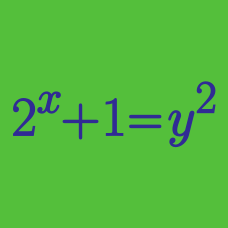Algebra

# Algebraic Manipulation: Level 1 Challenges

$\large{\color{#3D99F6}x = \sqrt\frac{\color{#D61F06}y - 1}{\color{#D61F06}y +1}}$
Express $y$ in terms of $x$.

$\Large\sqrt{3\sqrt{3\sqrt{3\cdots}}}=\sqrt{n} \quad, \quad n = \ ?$

$x=\sqrt { 2+\sqrt { 2+\sqrt { 2+\sqrt { 2\ldots } } } }$

What is the value of $x$?

$\huge{ x^{x^{x^{x^{x^{.^{.^.}}}}}}=2}$

Find the value of $x$ that satisfies the equation above.

$\large \sqrt{12+\sqrt{12+\sqrt{12+\sqrt{12 + ...}}}}= \ ?$

×